# JP2014119798A - Apparatus, program and method for estimating mobile target boarded by user carrying portable terminal - Google Patents

Apparatus, program and method for estimating mobile target boarded by user carrying portable terminal Download PDF

## Info

Publication number
JP2014119798A
JP2014119798A JP2012272316A JP2012272316A JP2014119798A JP 2014119798 A JP2014119798 A JP 2014119798A JP 2012272316 A JP2012272316 A JP 2012272316A JP 2012272316 A JP2012272316 A JP 2012272316A JP 2014119798 A JP2014119798 A JP 2014119798A
Authority
JP
Japan
Prior art keywords
base station
probability
time
movement
moving object
Prior art date
Legal status (The legal status is an assumption and is not a legal conclusion. Google has not performed a legal analysis and makes no representation as to the accuracy of the status listed.)
Granted
Application number
JP2012272316A
Other languages
Japanese (ja)
Other versions
JP6075725B2 (en
Inventor
Takafumi Watanabe

Mori Kurokawa

Shigeki Muramatsu

Original Assignee
Kddi Corp
Ｋｄｄｉ株式会社
Priority date (The priority date is an assumption and is not a legal conclusion. Google has not performed a legal analysis and makes no representation as to the accuracy of the date listed.)
Filing date
Publication date
Application filed by Kddi Corp, Ｋｄｄｉ株式会社 filed Critical Kddi Corp
Priority to JP2012272316A priority Critical patent/JP6075725B2/en
Publication of JP2014119798A publication Critical patent/JP2014119798A/en
Application granted granted Critical
Publication of JP6075725B2 publication Critical patent/JP6075725B2/en
Application status is Active legal-status Critical
Anticipated expiration legal-status Critical

• 238000004364 calculation methods Methods 0 abstract 5
• 230000002123 temporal effects Effects 0 abstract 3
• 239000010912 leaf Substances 0 abstract 2
• 239000000969 carrier Substances 0 abstract 1
• 230000001721 combination Effects 0 abstract 1
• 230000001276 controlling effects Effects 0 abstract 1
• 230000001788 irregular Effects 0 abstract 1

## Images

•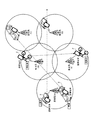•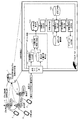•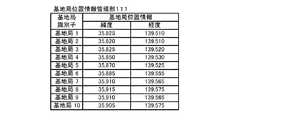•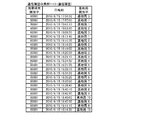•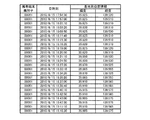•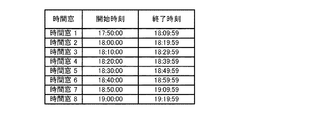•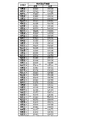•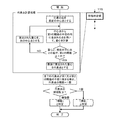•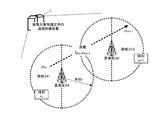•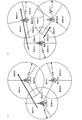•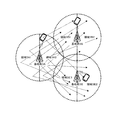•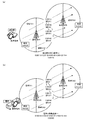•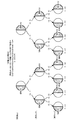•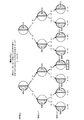## Abstract

PROBLEM TO BE SOLVED: To provide an apparatus and the like capable of estimating a mobile target boarded by a user carrying a portable terminal by using base-station positional information that can be obtained by a common carrier equipment, of which a spatial granularity is coarse, and of which a temporal interval is irregular, without requiring starting of the positioning function of the portable terminal.SOLUTION: An apparatus includes: movement probability calculation means for calculating a probability of movement, for all combinations, from individual division zones of a previous base station to individual division zones of a following base station; existence probability calculation means for controlling the movement probability calculation means so as to repeat the movement probability calculation means on the basis of a change of base stations connected to a portable terminal in accordance with a temporal elapse and generating, for each mobile target, a tree structure of a probability of existence using, as a node, a probability of movement in individual zone of the respective base stations in accordance with the temporal elapse; and mobile target estimation means for estimating a mobile target at a leaf of the tree structure generated by the existence probability calculation means, the leaf where the probability of existence is the highest, as the mobile target used by a user carrying the aforementioned portable terminal.

## Description

The present invention relates to a technique for estimating the movement of a user who has a mobile terminal.

In recent years, a mobile terminal represented by a mobile phone or a smartphone has generally been equipped with a positioning function such as GPS (Global Positioning System). Therefore, the user can measure the current position using the mobile terminal, and can receive various services by transmitting the position to the server via the network.

Conventionally, there is a technique in which a server estimates a moving object on which a user is boarded by collecting position information from a mobile terminal (see, for example, Non-Patent Document 1). According to this technique, it is possible to identify a moving object such as “car”, “train”, “airplane”, and “bicycle”. However, for the mobile terminal, it is necessary to always activate the GPS function and its application, and the amount of packets transmitted in the position information is also increased. As a result, the power consumption of the mobile terminal is increased, and the battery of the mobile terminal is consumed quickly.

On the other hand, there is also a technique for estimating a moving object using an acceleration sensor built in the portable terminal in order to keep the power consumption of the portable terminal low (for example, see Non-Patent Documents 2 and 3). In particular, according to the technique of Non-Patent Document 2, it is possible to estimate the moving object using the acceleration peak frequency or the like as a feature amount by utilizing the fact that the vibration pattern of acceleration differs for each moving object. However, the mobile terminal needs to transmit the measured acceleration data to the server. If the moving object on which the user is boarded is estimated in real time, the amount of packets of acceleration data to be transmitted increases as a result.

Leon Stenneth, Ouri Wolfson, Philip S. Yu, Bo Xu: Transportation ModeDetection using Mobile Phones and GIS Information.In Proceedings of the ACMSIGSPATIAL International Conference on Advances in Geographic Information Systems (2011) pp.54-63 Zhixian Yan, Vigneshwaran Subbaraju, DipanjanChakraborty, Archan Misra, KarlAberer: Energy-Efficient Continuous Activity Recognition on Mobile Phones: AnActivity-Adaptive Approach.In Proceedings of the International Symposium on Wearable Computing (2012) Arei Kobayashi, Shigeki Muramatsu, Daisuke Kamisaka, Takafumi Watanabe, Atsunori Minamikawa, Takeshi Iwamoto, Hiroyuki Yokoyama: Shaka: User Movement Estimation Considering Reliability, PowerSaving, and Latency Using Mobile Phone.IEICETransactions 94-D (6): 1153-1163 (2011 )

Usually, as a communication carrier equipment, it is possible to collect a history of position information of a base station under the control of the mobile terminal. Therefore, the inventors of the present application considered that it is preferable to estimate the moving object on which the user is boarded from only the base station position information. In this case, the mobile terminal does not need to activate the GPS function and does not need to transmit positioning information or acceleration data to the server. However, such base station position information has a problem that the spatial granularity is coarse and the time interval is not constant.

Therefore, in the present invention, the movement on which the user is boarded using the base station position information with a coarse spatial granularity and a non-constant time interval that can be acquired by the telecommunications carrier equipment without activating the positioning function of the mobile terminal. It is an object of the present invention to provide a communication equipment device, a program, and a method capable of estimating an object.

According to the present invention, it is an apparatus for estimating a moving object that is connected to a wide area wireless communication network and is carried by a user who has a mobile terminal,
For each portable terminal, communication history storage means for storing a plurality of communication histories in association with the date and time of communication and the base station position information;
Probability distribution accumulating means for accumulating the probability distribution of time t required for movement of distance l for each moving object;
An area dividing means for dividing and setting an area based on a predetermined radial distance centered on the base station in advance for each base station or for all base stations into k (k> 2) equal parts;
For each moving object, using the probability distribution of the probability distribution accumulating means, the movement probability calculating means for calculating the movement probability for all combinations from each divided area of the previous base station to each divided area of the subsequent base station;
Based on the change of the base station connected to the mobile terminal according to the passage of time, it is controlled to repeat the movement probability calculation means, and the movement probability of each divided region of each base station according to the passage of time is determined for each moving object. Existence probability calculating means for generating a tree structure of existence probability as a node;
The tree structure of the existence probability calculation means includes a movement object estimation means for estimating that the movement object in the leaf having the highest existence probability is being used by the user who possesses the portable terminal.

According to another embodiment of the device of the invention,
The movement probability calculation means
A plurality of arbitrary points are scattered in the previous divided area and the subsequent divided area, and the distance from the previous arbitrary point to the subsequent arbitrary point is connected to the previous base station and the subsequent base station. For each moving object, a movement probability that requires time t between the time of connection and the time of connection is calculated using a probability distribution,
It is also preferable to set the average value of the movement probabilities between a plurality of arbitrary points for each movement object as the movement probability that the movement object will be used from the previous divided area to the subsequent divided area.

According to another embodiment of the apparatus of the present invention, it is also preferable that the probability distribution of the probability distribution accumulating means is a probability distribution defined in advance by the average value ν of the moving speed and the standard deviation σ for each moving object. .

According to another embodiment of the apparatus of the present invention, the probability distribution is also preferably calculated by the following formula:
tn: Previous time
tn + 1: Later time
Ptn: Arbitrary first point at the previous time tn
Ptn + 1: Arbitrary second point at a later time tn + 1 l Ptn, Ptn + 1 : Distance between the first point Ptn and the second point Ptn + 1 g: From the first point Ptn Probability of moving to the second point Ptn + 1 at time tn + 1−tn

According to another embodiment of the device of the invention,
Time window dividing means for dividing the position information history generated by the position information history generating means into time windows (time intervals) of a predetermined time;
For each time window, when the probability distribution of the position based on the plurality of base station position information is multimodal, it further includes movement determination means for determining “movement”,
It is also preferable that the movement probability calculation means calculates the movement probability for a time window that is continuous for a predetermined number or more determined to be movement.

According to another embodiment of the device of the invention,
The movement determination means is for each time window.
(S1) An arbitrary point (position information) is set as the first center point,
(S2) Calculate the center of gravity from the center point using the points included in the circle with the first threshold radius,
(S3) Determine whether the difference between the center of gravity and the current center point is less than or equal to the second threshold,
(S4) If it is determined to be false by S3, the center of gravity is set as the next center point, the process returns to S2 again, and the process is repeated until the amount of change falls below the second threshold.
(S5) When determined to be true by S3, the center of gravity is used as representative point position information,
Finally, it is also preferable to determine “move” when there are a plurality of types of representative point position information for the time window.

According to another embodiment of the device of the invention,
Lastly, the stay movement determination means, when all the representative points are within the circumferential area of the radius of the first threshold value or the second threshold value for the time window, the number of types of converged representative points is one. It is also preferable to consider it to be.

According to the present invention, the above-described device is a communication equipment device connected to a wide area wireless communication network, and in order to store a communication history in a communication history storage means,
Base station location information management means for storing a base station identifier and base station location information in association with each other;
From a base station to which the mobile terminal is connected, a communication history collecting means for collecting a communication history of the date and time of communication for each mobile terminal and its base station identifier,
The apparatus further comprises position information history generation means for further associating the base station position information corresponding to the base station identifier for each communication history using the base station position information management means.

According to the present invention, there is provided a program for causing a computer mounted on an apparatus for estimating a moving object on which a user carrying a mobile terminal is connected to a wide area wireless communication network to function.
For each portable terminal, communication history storage means for storing a plurality of communication histories in association with the date and time of communication and the base station position information;
Probability distribution accumulating means for accumulating the probability distribution of time t required for movement of distance l for each moving object;
An area dividing means for dividing and setting an area based on a predetermined radial distance centered on the base station in advance for each base station or for all base stations into k (k> 2) equal parts;
For each moving object, using the probability distribution of the probability distribution accumulating means, the movement probability calculating means for calculating the movement probability for all combinations from each divided area of the previous base station to each divided area of the subsequent base station;
Based on the change of the base station connected to the mobile terminal according to the passage of time, it is controlled to repeat the movement probability calculation means, and the movement probability of each divided region of each base station according to the passage of time is determined for each moving object. Existence probability calculating means for generating a tree structure of existence probability as a node;
With respect to the tree structure of the existence probability calculating means, the computer is caused to function as a moving object estimation means for estimating that the user having the mobile terminal is using the moving object in the leaf having the highest existence probability. To do.

According to the present invention, a communication equipment connected to a wide area wireless communication network is a method for estimating a moving object on which a user carrying a mobile terminal is boarded,
For each portable terminal, a communication history storage unit that stores a plurality of communication histories in association with the date and time of communication and the base station position information,
For each moving object, a probability distribution accumulating unit that accumulates a probability distribution of time t required for movement of distance l,
For each base station or for all base stations, an area dividing unit that preliminarily divides and sets an area based on a predetermined radial distance centered on the base station into k (k> 2) equal parts,
For the communication history stored in the communication history storage unit, for each moving object, using the probability distribution of the probability distribution storage means, all combinations from each divided region of the previous base station to each divided region of the subsequent base station A first step of calculating a movement probability for
Based on the change of the base station connected to the mobile terminal according to the passage of time, it is controlled to repeat the movement probability calculation means, and the movement probability of each divided region of each base station according to the passage of time is determined for each moving object. A second step of generating a tree structure of existence probabilities as nodes;
The tree structure of the second step includes a third step of estimating that the moving object in the leaf having the highest existence probability is being used by the user who possesses the mobile terminal.

According to the communication equipment apparatus, program, and method of the present invention, the base station location information with a coarse spatial granularity and a non-constant time interval that can be acquired by the communication carrier equipment without activating the positioning function of the mobile terminal. It is possible to estimate the moving object on which the user is boarded.

It is a spatial external view showing the movement of a moving object. It is a functional block diagram of the apparatus which estimates the moving target object in this invention. It is a table | surface of base station position information. It is a table | surface of communication history. It is the table | surface which matched base station position information with the communication history. It is a table | surface of each time window. It is the table | surface which divided | segmented the table | surface of FIG. 5 by the time window of FIG. It is a flowchart for determining whether it is multimodal. It is explanatory drawing showing the distance which moved between division areas. It is explanatory drawing showing the division area of k = 3, 4. FIG. It is explanatory drawing showing scattering of the arbitrary points for calculating the movement probability between division areas. It is explanatory drawing showing the movement probability in a motor vehicle and a train for each line segment which connects an arbitrary point from the previous division area to the subsequent division area. It is explanatory drawing showing the tree structure of the existence probability according to time progress about the moving target object "automobile." It is explanatory drawing showing the tree structure of the existence probability according to time progress about the moving target object "train."

Hereinafter, embodiments of the present invention will be described in detail with reference to the drawings.

FIG. 1 is a spatial external view showing movement of a moving object.

A mobile terminal (for example, a mobile phone or a smartphone) possessed by a user is always under the control of a base station and continues to communicate with the base station regardless of the position. According to FIG. 1, the user is on the moving object, and the movement of the mobile terminal is the same as the movement of the moving object.

In a carrier equipment that integrates a large number of base stations, base station position information with a coarse spatial granularity and a constant time interval can always be collected for each mobile terminal. “Coarse spatial granularity” means that the geographical distance between location information is relatively long. Further, “the time interval is not constant” means that the acquisition time intervals of the position information are relatively varied.

The base station 3 connected to the wide area wireless communication network (mobile phone network) acquires the date and time as a communication history by communicating with the mobile terminal 2 located under the base station 3. The communication history is not limited to the time of transmission / reception of a mail requiring a user operation on the mobile terminal or browsing of a web page. The base station 3 also obtains a communication history from the portable terminal 2 when transmitting and receiving data automatically executed by an application installed in the portable terminal.

According to FIG. 1, “train”, “bicycle”, and “automobile” are represented as moving objects. For each moving object, the communication history of the base station changes as follows with the passage of time.
"Train": Base station 3A-> Base station 3B-> Base station 3C
“Bicycle”: Base station 3B-> Base station 3E
"Automobile": Base station 3A-> Base station 3B-> Base station 3D

FIG. 2 is a functional configuration diagram of an apparatus for estimating a moving object in the present invention.

The device 1 according to the present invention estimates a moving object on which a user carrying a mobile terminal is boarded, and accumulates a communication history in advance. Further, the device 1 may be a communication equipment device that collects communication histories from the base station 3 by being installed in a wide area wireless communication network (mobile phone network) without storing the communication histories in advance.

According to FIG. 2, the apparatus 1 for moving object estimation includes, as characteristic features of the present invention, a communication history accumulation unit 120, a probability distribution accumulation unit 121, a region division unit 122, a movement probability calculation unit 123, The existence probability calculation unit 124, the moving object estimation unit 125, and the application processing unit 13 are included. The application processing unit 13 executes various services based on the moving object on which each user is estimated estimated according to the present invention. These functional components excluding the communication interface unit are realized by executing a program that causes a computer installed in the apparatus to function. In addition, according to FIG. 2, the flow of processing using each functional component can be understood as a method for estimating a moving object.

In addition, when the device 1 is a communication facility device installed in a wide area wireless communication network (mobile phone network), the apparatus 1 is connected to the wide area communication network as an optional function in order to accumulate communication history in the communication history accumulation unit 121. The communication interface unit 10, the base station location information management unit 111, the communication history collection unit 112, and the location information history generation unit 113 are further included. It is also preferable to further include a time window dividing unit 114 and a movement determining unit 115 for the communication history output from the communication history storage unit 120. Below, the apparatus 1 is demonstrated as what is a communication equipment apparatus.

[Base station location information management unit 111]
Base station location information management section 111 stores a base station identifier and base station location information in association with each other.

FIG. 3 is a table of base station position information. For each base station identifier, latitude / longitude base station position information is associated. According to FIG. 3, it can be understood that the base station 1 is installed at a position of latitude 35.825 and longitude 139.510. Further, it can be understood that the base station 3 is installed at a position of latitude 35.825 and longitude 139.520. Such base station position information may be stored in advance in the base station position information management unit 111 or may be acquired from each base station 3 via the communication interface unit 10. Good.

[Communication history collection unit 112]
The communication history collection unit 112 collects the communication history of the date and time and the base station identifier for each mobile terminal 2 from the base station 3 to which the mobile terminal 2 is connected.

FIG. 4 is a communication history table. The communication history is a record in which the base station 3 accepts communication from the mobile terminal 2. In the communication history, “date and time” and “base station identifier” are associated with each “terminal identifier” (address, telephone number, identification number, etc.) of the mobile terminal 2 as follows.
Communication history (terminal identifier, date / time, base station identifier)
According to the first log in FIG. 4, it is recorded that the mobile terminal 0001 communicated with the base station 3 on June 15, 2010 at 17:54:50. Further, it is recorded that the mobile terminal 0001 communicated with the base station 1 on June 15, 2010 at 17:57:00.

[Position information history generation unit 113]
The location information history generation unit 113 further associates base station location information corresponding to the base station identifier for each communication history using the base station location information management unit 111. The communication history is output to the communication history storage unit 120.

FIG. 5 is a table in which the base station position information is associated with the communication history. In the table of FIG. 5, the latitude and longitude of the base station of FIG. 3 are associated with the base station identifier portion of the table of FIG. 4. According to the first log in FIG. 5, it can be understood that the mobile terminal 0001 communicated with the base station at latitude 35.825 and longitude 139.520 on June 15, 2010, 17:54:50. Further, it can be understood that the portable terminal 0001 communicated with the base station at latitude 35.825 and longitude 139.510 at 17:57:00 on June 15, 2010.

[Communication history storage unit 120]
The communication history accumulation unit 120 accumulates the communication history output from the position information history generation unit 113.

[Time window dividing unit 114]
The time window dividing unit 114 divides the plurality of communication histories output from the communication history storage unit 120 into predetermined time windows (time intervals). The time window is determined by the time width T and the shift width S. The shift width S is obtained by delaying the start time by S. That is, when T> S, the time window is overlapped by T−S.

The time width T is a parameter that determines “with which time width it is determined to move”. When there is no predetermined number or more of position information within the time window, it is difficult to determine stay / movement. For this purpose, the time width T needs to be determined based on the total number of communication histories so that as much position information as possible falls within the time window as much as possible.

Further, when the shift width S is shortened, the time resolution of the stay time section is increased. On the other hand, if the width is increased, the number of time windows increases, and the amount of calculation increases. Therefore, the shift width S needs to be determined according to the time resolution and processing time required by the application.

FIG. 6 is a table for each time window. FIG. 6 shows the start time and end time of each time window when T = 20 minutes and S = 10 minutes. According to FIG. 6, the time window 1 is 17:50:00 to 18:09:59, and T = 00: 20: 00. The time window 2 is 18:00:00 to 18:19:59, and S = 00: 10: 00 with respect to the time window 1.

FIG. 7 is a table obtained by dividing the table of FIG. 5 by the time window of FIG. According to FIG. 7, five communication histories are recorded in the time window 1, and eight communication histories are recorded in the time window 3.

[Movement determination unit 115]
For each time window, the movement determination unit 115 determines that the position probability distribution based on the plurality of base station position information is multimodal, and otherwise determines “stay”. To do. “Multimodal” means that the probability distribution of positions has a plurality of mountain shapes. That is, being multimodal for one time window (for example, 20 minutes) can be determined as “moving” for that position. On the contrary, when the probability distribution of the position has the shape of one mountain, it means unimodality. Being unimodal for one time window can be determined to be “staying” for that location.

Note that the movement determination unit 115 preferably sets “not determined” for a time window in which a predetermined number or more of communication histories are not recorded for the time clustering unit 128 described later. For example, a time window in which a predetermined number of two or more communication histories are not recorded is set as “undecided”.

FIG. 8 is a flowchart for determining whether or not there is multimodality.

According to FIG. 8, the movement determination unit 115 executes a representative point calculation process in order to determine whether or not the probability distribution of the position information is multimodal. As a result, if there are a plurality of types of representative points, it is determined to be multimodal and “moving”, and if the number of types of representative points is 1, it is unimodal and “staying”. Determined.

The representative point calculation process is executed by the following steps for a plurality of pieces of position information included in each time window.
(S1) An arbitrary point (position information) is set as the first center point.
(S2) The center of gravity is calculated from the center point using a point (position information) included in a circle having a radius of the first threshold (for example, 2 km).
(S3) Next, it is determined whether or not the difference (change amount) between the center of gravity and the current center point is equal to or less than a second threshold value (for example, 100 m).
(S4) If determined to be false by S3, the center of gravity is set as the next center point. And it returns to S2 again and repeats until the variation | change_quantity falls below the 2nd threshold value.
(S5) If determined to be true by S3, the center of gravity (the converged point) is set as the representative point.
Finally, for each time window, if the number of converged representative points is one, it is determined as “stay”, and if there are a plurality of types, it is determined as “moving”. Note that when all the converged representative points are within the circumferential region having the radius of the second threshold, the number of converged representative points may be regarded as one. Here, regarding the time window, when all the representative points fall within the circumferential region having the radius of the first threshold value or the second threshold value, it is considered that the number of types of converged representative points is one. Is also preferable.

Hereinafter, the stay / movement is specifically determined for the time window 1 and the time window 3 in FIG. 7 using the representative point extraction process.

(Movement judgment in time window 1)
(1) The first centroid for the point (35.825, 139.52) in time window 1 is calculated. Since the distances between the points of time window 1 (35.825, 139.52) and the other points included in time window 1 are all within 2 km, the average of all other points is (35.824, 139.514).
(2) Calculate the second center of gravity for the point (35.825, 139.52) of time window 1. In the second centroid calculation, the center point is (35.824, 139.514). Since the distance between the center point and the other points included in the time window 1 is all within 2 km, the average of all the other points is (35.824, 139.514).
(3) Since the results of the first and second centroid calculations are the same and the amount of change is 100 m or less, the representative points of the time window 1 points (35.825, 139.52) are (35.824, 139.514).
(4) Calculate the first center of gravity for the point (35.825, 139.51) of time window 1. Since the distances between the points of time window 1 (35.825, 139.510) and the other points included in time window 1 are all within 2 km, the average of all the other points is (35.824, 139.514).
(5) Calculate the second center of gravity for the point (35.825, 139.51) of time window 1. In the second centroid calculation, the center point is (35.824, 139.514). Since the distance between the center point and the other points included in the time window 1 is all within 2 km, the average of all the other points is (35.824, 139.514).
(6) The results of the first and second centroid calculations are the same and the amount of change is 100 m or less, so the representative points of the time window 1 points (35.825, 139.51) are (35.824, 139.514).
(7) Calculate the first centroid for the point (35.820, 139.51) in time window 1. Since the distances between the points of time window 1 (35.820, 139.510) and the other points included in time window 1 are all within 2 km, the average of all other points is (35.824, 139.514).
(8) Calculate the second center of gravity for the point (35.820, 139.51) of time window 1. In the second centroid calculation, the center point is (35.824, 139.514). Since the distance between the center point and the other points included in the time window 1 is all within 2 km, the average of all the other points is (35.824, 139.514).
(9) Since the results of the first and second centroid calculations are the same, and the amount of change is 100 m or less, the representative points of the time window 1 points (35.820, 139.51) are (35.824, 139.514).
From the above, the calculated representative points are (35.824, 139.514) for any point, and the number of types of representative points is 1, so that the time window 1 is determined as “stay”.

(Movement judgment in time window 3)
(1) The first centroid about the point (35.825, 139.51) of the time window 3 is calculated. When calculating the distance between the points in time window 3 (35.825, 139.510) and the other points in time window 3, (35.825, 139.51), (35.820, 139.51), (35.820, 139.51), (35.820, 139.51) is within 2 km, but other than it is over 2 km, so taking the average of the above points gives (35.822, 139.512).
(2) Calculate the second center of gravity for the point (35.825, 139.51) of time window 3. In the second centroid calculation, the center point is (35.822, 139.512). The distance between the center point and the other points included in the time window 3 is calculated as (35.825, 139.51), (35.820, 139.51), (35.820, 139.51), (35.825, 139.52). ), (35.820, 139.51) are within 2 km but other than 2 km, the average of the above points is (35.822, 139.512).
(3) The results of the first and second centroid calculations are the same, and the amount of change is 100 m or less, so the representative points of the time window 3 points (35.825, 139.51) are (35.822, 139.512).
(4) The first centroid about the point (35.850, 139.53) of the time window 3 is calculated. When calculating the distance between the point (35.850, 139.530) of time window 3 and the other points included in time window 3, (35.850, 139.53) and (35.850, 139.53) are within 2 km, but otherwise, it exceeds 2 km The average of the above points is (35.850, 139.53).
(5) Calculate the second center of gravity for the point (35.850, 139.53) in time window 3. In the second centroid calculation, the center point is (35.850, 139.53). When the distance between the center point and other points included in the time window 3 is calculated, (35.850, 139.53) and (35.850, 139.53) are within 2 km, but otherwise 2 km. Therefore, the average of the above points is (35.850, 139.53).
(6) The results of the first and second centroid calculations are the same and the amount of change is 100 m or less, so the representative points of the time window 3 points (35.850, 139.53) are (35.850, 139.53).
(7) Calculation of representative points of other points in the time window 3 is omitted.
As described above, since the calculated representative points are (35.822, 139.512), (35.850, 139.53) (hereinafter omitted) and two or more, the time window 3 is determined to be “moving”.

In addition, it is preferable that the movement determination unit 115 determines whether or not the plurality of base station position information is unimodal by using kernel density estimation for each base station position information. Kernel density estimation is a method of extrapolating data from a sample of a population in order to estimate a probability density function of a random variable. According to this method, a hump that becomes a peak can be derived using a kernel function.

[Probability distribution storage unit 121]
The probability distribution accumulating unit 121 accumulates the probability distribution of the time t required for the movement of the distance l for each moving object. The probability distribution is calculated for each moving object by the following formula defined in advance by the average value ν of the moving speed and the standard deviation σ.
tn: Previous time
tn + 1: Later time
Ptn: Arbitrary first point at the previous time tn
Ptn + 1: Arbitrary second point at a later time tn + 1 l Ptn, Ptn + 1 : Distance between the first point Ptn and the second point Ptn + 1 g: From the first point Ptn Probability of moving to the second point Ptn + 1 at time tn + 1−tn

[Area Dividing Unit 122]
The area dividing unit 122 divides an area (for example, a circular area) based on a predetermined radius distance r around the base station into k (k> 2) equal parts for each base station or for all base stations. To set.

FIG. 9 is an explanatory diagram showing the distance moved between the divided areas.

According to FIG. 9, the circular area based on the predetermined radial distance r centered on the base station is divided into 2 (k = 2) equal parts. Here, the moving object located at the first point Ptn (region 3A1) at time tn has moved to the second point Ptn + 1 (region 3B1) at time tn + 1. The distance is represented by l Ptn, Ptn + 1 .

FIG. 10 is an explanatory diagram showing the divided areas of k = 3 and 4.

In FIG. 10A, a circular area based on a predetermined radial distance r centered on the base station is divided into 3 (k = 3) equal parts. In FIG. 10B, a circular area based on a predetermined radial distance r centered on the base station is divided into 4 (k = 4) equal parts.

Note that the angle θ of the divided region based on the equal division k may be adaptively changed. According to FIG. 10, a straight line connecting the first point Ptn and the second point Ptn + 1 is used as a reference line, and is divided into a plurality of regions.

Further, the predetermined distance radius r may be adaptively changed. For example, when the radius is r at time tn, the radius r × α (α> 1) is set at time tn + 1. For example, when Δtn = tn + 1−tn, α increases in proportion to Δtn.

[Movement probability calculation unit 123]
For each moving object, the movement probability calculation unit 123 uses the probability distribution of the probability distribution storage unit 121 to calculate the movement probabilities for all combinations from each divided region of the previous base station to each divided region of the subsequent base station. calculate. For example, in the case of FIG. 9, for each moving object, the probability of moving from the first point Ptn of the region 3A1 at time tn to the second point Ptn + 1 of the region 3B2 at time tn + 1 is calculated. This utilizes the fact that the probability distribution of the moving speed differs for each moving object.

FIG. 11 is an explanatory diagram showing scattering of arbitrary points for calculating the movement probability between the divided regions.

According to FIG. 11, the movement probability calculation unit 123 scatters a plurality of arbitrary points on the previous divided area and the subsequent divided area. Then, with respect to the distance l from any previous point to any subsequent point, the movement probability that requires time t between the time connected to the previous base station and the time connected to the subsequent base station is moved. For each object, calculation is performed using a probability distribution.

FIG. 12 is an explanatory diagram showing movement probabilities in a car and a train for each line segment connecting arbitrary points from the previous divided area to the subsequent divided area.

According to FIG. 12, four arbitrary points are scattered in each of the region 3A1 and the region 3B2, and four line segments are represented by broken-line arrows. The distance between the previous time tn = 9: 00: 20 and the later time tn + 1 = 9: 05: 20 depends on the speed of the moving object. Here, it is assumed that the moving speed of each moving object follows a probability distribution. By using the probability distribution of the movement speed accumulated in the movement probability accumulation unit 121, the movement probability is calculated from the movement time and the distance.

According to FIG. 12A, it is assumed that the moving speed of the object “automobile” is an average value ν = 30 km / h and a standard deviation σ = √350. The movement probability follows a probability distribution N (30 km, 350). Assuming that the distance of the line segment 1 is 3 km, the moving probability that the moving object “car” would have moved is as follows.
≒ 0.00112 (However, Δt = 1/3600)
Assuming that the distance of the line segment 2 is 3.2 km, the movement probability that would have been moved by the moving object “car” is 0.00142 according to the above formula.
Assuming that the distance of the line segment 3 is 3.5 km, the movement probability that would have been moved by the moving object “automobile” is 0.00186 according to the above formula.
Assuming that the distance of the line segment 4 is 3.2 km, the movement probability that would have been moved by the moving object “car” is 0.00142 according to the above formula.

According to FIG. 12B, it is assumed that the average value ν = 40 km / h and the standard deviation σ = 2 for the moving speed of the object “train”. The movement probability follows a probability distribution N (40 km, 400). Assuming that the distance of the line segment 1 is 3 km, the movement probability that would have been moved by the moving object “train” is as follows.
≒ 0.00243 (However, Δt = 1/3600)
Assuming that the distance of the line segment 2 is 3.2 km, the movement probability that would have been moved by the moving object “train” is 0.00247 according to the above formula.
If the distance is 3.5 km for the line segment 3, the movement probability that would have been moved by the moving object “train” is 0.00147 according to the above formula.
Assuming that the distance of the line segment 4 is 3.2 km, the movement probability that would have been moved by the moving object “train” is 0.00142 according to the above formula.

Next, for each moving object, the average value of the moving probability of all line segments (between arbitrary points) is moved from the previous divided area to the subsequent divided area. According to FIG. 12A, the movement probability that the moving object “automobile” moves from the region 3A1 to the region 3B2 is calculated by the following equation.
(0.00112 + 0.00142 + 0.00188 + 0.00142) /4=0.00146
According to FIG. 12B, the movement probability that the moving object “train” moves from the region 3A1 to the region 3B2 is calculated by the following equation.
(0.00243 + 0.00247 + 0.00147 + 0.00245) /4=0.00220

In addition, according to FIG. 2 mentioned above, the movement probability calculation part 123 will calculate a movement probability about the time window which continues more than the predetermined number determined as the movement by the movement determination part 115. FIG. That is, when the moving object is “stay” (stop), the movement probability is not calculated.

[Existence probability calculation unit 124]
The existence probability calculation unit 124 controls to repeat the movement probability calculation means based on the change of the base station connected to the mobile terminal according to the passage of time. Then, for each moving object, a tree structure of existence probabilities is generated with the movement probability of each divided region of each base station corresponding to the passage of time as a node.

FIG. 13 is an explanatory diagram showing a tree structure of existence probabilities according to the passage of time for the moving object “automobile”.
FIG. 14 is an explanatory diagram showing a tree structure of existence probabilities according to the passage of time for the moving object “train”.

FIG. 13 and FIG. 14 show a case where the mobile terminal 2 moves as base stations 3A-> 3B-> 3C as time elapses at times tn, tn + 1, tn + 2. At this time, the movement probabilities are recorded as histories on the branches of the tree structure for all combinations between the previous divided area and the subsequent divided area. Finally, the product of the movement probabilities until time tn + 2 is the cumulative movement probability of the tree-structured leaves.

It should be noted that although the tree structure becomes deeper as time elapses, it is preferable to discard a node whose cumulative probability is equal to or less than a predetermined threshold from the viewpoint of securing a data storage area.

[Movement object estimation unit 125]
With respect to the tree structure of the existence probability calculation unit 124, it is estimated that the moving object in the leaf having the highest existence probability (cumulative probability) is used by the user who possesses the portable terminal.

According to FIG. 13 and FIG. 14, the leaf having the highest existence probability is a leaf having the existence probability of 0.24 following the region 3A1-> 3B2-> 3C2 in FIG. This is the moving object “train”. Therefore, it can be estimated that the user who possesses the portable terminal would have boarded the “train” at times tn to tn + 2.

As described above in detail, according to the communication equipment device, program, and method of the present invention, the spatial granularity and time that can be acquired by the communication carrier equipment without activating the positioning function of the mobile terminal is large. It is possible to estimate a moving object on which the user is boarded using base station position information whose intervals are not constant.

Various changes, modifications, and omissions of the above-described various embodiments of the present invention can be easily made by those skilled in the art. The above description is merely an example, and is not intended to be restrictive. The invention is limited only as defined in the following claims and the equivalents thereto.

DESCRIPTION OF SYMBOLS 1 Communication equipment 10 Communication interface part 111 Base station location information management part 112 Communication history collection part 113 Location information history generation part 114 Time window division part 115 Movement determination part 120 Communication history accumulation part 121 Probability distribution accumulation part 122 Area division part 123 Movement probability calculation unit 124 Presence probability calculation unit 125 Moving object estimation unit 13 Application processing unit 2 Portable terminal 3 Base station

## Claims (10)

1. A device that is connected to a wide area wireless communication network and estimates a moving object on which a user carrying a mobile terminal is boarded,
For each portable terminal, communication history storage means for storing a plurality of communication histories in association with the date and time of communication and the base station position information;
Probability distribution accumulating means for accumulating the probability distribution of time t required for movement of distance l for each moving object;
An area dividing means for dividing and setting an area based on a predetermined radial distance centered on the base station in advance for each base station or for all base stations into k (k> 2) equal parts;
For each moving object, using the probability distribution of the probability distribution accumulating means, the movement probability calculating means for calculating the movement probability for all combinations from each divided area of the previous base station to each divided area of the subsequent base station. When,
Control to repeat the movement probability calculation means based on the change of the base station connected to the mobile terminal according to the passage of time, for each moving object, for each divided region of each base station according to the passage of time An existence probability calculating means for generating a tree structure of an existence probability having a movement probability as a node;
The tree structure of the existence probability calculating means includes a moving object estimation means for estimating that a user having the mobile terminal is using a moving object in a leaf having the highest existence probability. apparatus.
2. The movement probability calculation means includes
A plurality of arbitrary points are scattered in the previous divided area and the subsequent divided area, and the distance from the previous arbitrary point to the subsequent arbitrary point is connected to the previous base station and the subsequent base station. For each moving object, the movement probability that requires time t between the time of connection and the time calculated using the probability distribution is calculated,
For each moving object, an average value of moving probabilities between a plurality of arbitrary points is set as a moving probability that the moving object will be used from the previous divided area to the subsequent divided area. The apparatus according to claim 1.
3.   3. The apparatus according to claim 1, wherein the probability distribution of the probability distribution accumulating unit is a probability distribution defined in advance by an average value ν and a standard deviation σ of moving speed for each moving object.
4. The probability distribution is calculated by the following formula:
tn: Previous time
tn + 1: Later time
Ptn: Arbitrary first point at the previous time tn
Ptn + 1: Arbitrary second point at a later time tn + 1 l Ptn, Ptn + 1 : Distance between the first point Ptn and the second point Ptn + 1 g: From the first point Ptn The device according to claim 3, characterized in that it has a probability of moving to the second point Ptn + 1 at time tn + 1-tn.
5. Time window dividing means for dividing the position information history generated by the position information history generating means into time windows (time intervals) of a predetermined time;
For each time window, there is further provided a movement determination means for determining that the position probability distribution based on the plurality of base station position information is multimodal when the position probability distribution is multimodal,
The apparatus according to any one of claims 1 to 4, wherein the movement probability calculation means calculates the movement probability for a predetermined number of time windows that are determined to move.
6. The movement determination means is provided for each time window.
(S1) An arbitrary point (position information) is set as the first center point,
(S2) Calculate the center of gravity from the center point using the points included in the circle with the first threshold radius,
(S3) Determine whether the difference between the center of gravity and the current center point is less than or equal to the second threshold,
(S4) If it is determined to be false by S3, the center of gravity is set as the next center point, the process returns to S2 again, and the process is repeated until the amount of change falls below the second threshold.
(S5) When determined to be true by S3, the center of gravity is used as representative point position information,
6. The apparatus according to claim 5, wherein when there are a plurality of types of representative point position information for the time window, it is determined as “move”.
7.   Lastly, the stay movement determination means has one converged representative point type number when all the representative points fall within the circumferential area of the radius of the first threshold value or the second threshold value for the time window. The device according to claim 6, characterized in that
8. A communication facility apparatus in which the apparatus according to any one of claims 1 to 7 is connected to a wide-area wireless communication network, in order to store a communication history in the communication history storage unit.
Base station location information management means for storing a base station identifier and base station location information in association with each other;
From a base station to which the mobile terminal is connected, a communication history collecting means for collecting a communication history of the date and time of communication for each mobile terminal and its base station identifier,
A communication equipment apparatus, further comprising position information history generation means for further associating the base station position information corresponding to the base station identifier for each communication history using the base station position information management means.
9. A program that is connected to a wide area wireless communication network and that functions as a computer mounted on a device that estimates a moving object on which a user carrying a mobile terminal is boarded,
For each portable terminal, communication history storage means for storing a plurality of communication histories in association with the date and time of communication and the base station position information;
Probability distribution accumulating means for accumulating the probability distribution of time t required for movement of distance l for each moving object;
An area dividing means for dividing and setting an area based on a predetermined radial distance centered on the base station in advance for each base station or for all base stations into k (k> 2) equal parts;
For each moving object, using the probability distribution of the probability distribution accumulating means, the movement probability calculating means for calculating the movement probability for all combinations from each divided area of the previous base station to each divided area of the subsequent base station. When,
Control to repeat the movement probability calculation means based on the change of the base station connected to the mobile terminal according to the passage of time, for each moving object, for each divided region of each base station according to the passage of time An existence probability calculating means for generating a tree structure of an existence probability having a movement probability as a node;
For the tree structure of the existence probability calculating means, the computer is caused to function as a moving object estimating means for estimating that a moving object in a leaf having the highest existence probability is being used by a user having the portable terminal. Program.
10. For a communication equipment connected to a wide area wireless communication network, a method for estimating a moving object on which a user carrying a mobile terminal is boarded,
For each portable terminal, a communication history storage unit that stores a plurality of communication histories in association with the date and time of communication and the base station position information,
For each moving object, a probability distribution accumulating unit that accumulates a probability distribution of time t required for movement of distance l,
For each base station or for all base stations, an area dividing unit that preliminarily divides and sets an area based on a predetermined radial distance centered on the base station into k (k> 2) equal parts,
For the communication history stored in the communication history storage unit, for each moving object, using the probability distribution of the probability distribution storage means, from each divided region of the previous base station to each divided region of the subsequent base station A first step of calculating movement probabilities for all combinations;
Control to repeat the movement probability calculation means based on the change of the base station connected to the mobile terminal according to the passage of time, for each moving object, for each divided region of each base station according to the passage of time A second step of generating a tree structure of existence probabilities having a movement probability as a node;
And a third step of estimating that the user having the mobile terminal is using the moving object in the leaf having the highest existence probability for the tree structure in the second step.
JP2012272316A 2012-12-13 2012-12-13 Apparatus, program, and method for estimating moving object on which user having portable terminal boarding Active JP6075725B2 (en)

## Priority Applications (1)

Application Number Priority Date Filing Date Title
JP2012272316A JP6075725B2 (en) 2012-12-13 2012-12-13 Apparatus, program, and method for estimating moving object on which user having portable terminal boarding

## Applications Claiming Priority (1)

Application Number Priority Date Filing Date Title
JP2012272316A JP6075725B2 (en) 2012-12-13 2012-12-13 Apparatus, program, and method for estimating moving object on which user having portable terminal boarding

## Publications (2)

Publication Number Publication Date
JP2014119798A true JP2014119798A (en) 2014-06-30
JP6075725B2 JP6075725B2 (en) 2017-02-08

# Family

## Family Applications (1)

Application Number Title Priority Date Filing Date
JP2012272316A Active JP6075725B2 (en) 2012-12-13 2012-12-13 Apparatus, program, and method for estimating moving object on which user having portable terminal boarding

## Country Status (1)

JP (1) JP6075725B2 (en)

## Cited By (5)

* Cited by examiner, † Cited by third party
Publication number Priority date Publication date Assignee Title
JP2015170144A (en) * 2014-03-07 2015-09-28 Kddi株式会社 Device, program and method for estimating place of stay of user carrying around mobile terminal
JP2016134731A (en) * 2015-01-19 2016-07-25 株式会社Ｋｄｄｉ研究所 Device, program, and method for estimating used route from communication history
JP2016133488A (en) * 2015-01-22 2016-07-25 株式会社Ｋｄｄｉ研究所 Device, program and method for estimating travel speed using plural estimation distances
JP2017143472A (en) * 2016-02-12 2017-08-17 Kddi株式会社 Device, program and method for estimating user's movement object
JP2017219466A (en) * 2016-06-09 2017-12-14 株式会社デンソー Radar system

## Citations (7)

* Cited by examiner, † Cited by third party
Publication number Priority date Publication date Assignee Title
JP2001167378A (en) * 1999-12-10 2001-06-22 Matsushita Electric Ind Co Ltd Method and device for estimating road traffic congestion
JP2004228781A (en) * 2003-01-21 2004-08-12 Ntt Docomo Inc Location deciding method, mobile communication system, and location deciding apparatus
JP2006012144A (en) * 2003-02-25 2006-01-12 Matsushita Electric Ind Co Ltd Mobile terminal
JP2008152655A (en) * 2006-12-19 2008-07-03 Ntt Docomo Inc Information service provision system, object behavior estimation apparatus and object behavior estimation method
JP2012085095A (en) * 2010-10-12 2012-04-26 Kddi Corp Device, program, and method for estimating significant area of user holding portable terminal
JP2014116808A (en) * 2012-12-10 2014-06-26 Kddi Corp Device, program and method for estimating staying place of user carrying portable terminal
JP2014191531A (en) * 2013-03-27 2014-10-06 Kddi Corp Device, program and method for estimating place of short-time stay of user carrying mobile terminal

## Patent Citations (7)

* Cited by examiner, † Cited by third party
Publication number Priority date Publication date Assignee Title
JP2001167378A (en) * 1999-12-10 2001-06-22 Matsushita Electric Ind Co Ltd Method and device for estimating road traffic congestion
JP2004228781A (en) * 2003-01-21 2004-08-12 Ntt Docomo Inc Location deciding method, mobile communication system, and location deciding apparatus
JP2006012144A (en) * 2003-02-25 2006-01-12 Matsushita Electric Ind Co Ltd Mobile terminal
JP2008152655A (en) * 2006-12-19 2008-07-03 Ntt Docomo Inc Information service provision system, object behavior estimation apparatus and object behavior estimation method
JP2012085095A (en) * 2010-10-12 2012-04-26 Kddi Corp Device, program, and method for estimating significant area of user holding portable terminal
JP2014116808A (en) * 2012-12-10 2014-06-26 Kddi Corp Device, program and method for estimating staying place of user carrying portable terminal
JP2014191531A (en) * 2013-03-27 2014-10-06 Kddi Corp Device, program and method for estimating place of short-time stay of user carrying mobile terminal

## Cited By (6)

* Cited by examiner, † Cited by third party
Publication number Priority date Publication date Assignee Title
JP2015170144A (en) * 2014-03-07 2015-09-28 Kddi株式会社 Device, program and method for estimating place of stay of user carrying around mobile terminal
JP2016134731A (en) * 2015-01-19 2016-07-25 株式会社Ｋｄｄｉ研究所 Device, program, and method for estimating used route from communication history
JP2016133488A (en) * 2015-01-22 2016-07-25 株式会社Ｋｄｄｉ研究所 Device, program and method for estimating travel speed using plural estimation distances
JP2017143472A (en) * 2016-02-12 2017-08-17 Kddi株式会社 Device, program and method for estimating user's movement object
JP2017219466A (en) * 2016-06-09 2017-12-14 株式会社デンソー Radar system
WO2017213199A1 (en) * 2016-06-09 2017-12-14 株式会社デンソー Radar device and method for detecting object by radar device

## Also Published As

Publication number Publication date
JP6075725B2 (en) 2017-02-08

## Similar Documents

Publication Publication Date Title
Mohan et al. Nericell: rich monitoring of road and traffic conditions using mobile smartphones
US8700069B2 (en) Systems and methods for mobile terminal location determination using radio signal parameter measurements
CA2710842C (en) Providing wi-fi location information to a mobile device in order to estimate its position
Michalevsky et al. Powerspy: Location tracking using mobile device power analysis
US8634853B2 (en) Method for enhancing location identity through incorporation of shorter-range communication and sensing (nearlocate)
CN102066969B (en) Method and device for location determination
US9401086B2 (en) Automatic update of crowd and traffic data using device monitoring
JP2014524167A (en) Indoor floor and location estimation using a three-step process
KR20130120366A (en) Hybrid mobile phone geopositioning
US8229473B1 (en) Geo-fence
JP2016105620A (en) Method and device for analyzing user traffic in predetermined region
US8542637B2 (en) Clustering crowd-sourced data for determining beacon positions
US9026094B2 (en) Method and apparatus for use of performance history data in positioning method selection
US8849312B2 (en) User description based on contexts of location and time
US9374661B2 (en) Travel pattern discovery using mobile device sensors
US8618984B2 (en) Selecting beacons for location inference
Liu et al. Improving positioning accuracy using GPS pseudorange measurements for cooperative vehicular localization
Namiot et al. Geofence and network proximity
Hoh et al. Achieving guaranteed anonymity in gps traces via uncertainty-aware path cloaking
US9179435B2 (en) Filtering and clustering crowd-sourced data for determining beacon positions
JP5653647B2 (en) Predicting the presence of mobile user equipment
JP2012085095A (en) Device, program, and method for estimating significant area of user holding portable terminal
US20150365796A1 (en) A method of generating an alert message
CN103997757A (en) Pseudo base station locating method and device and information reporting method and device
CN104823465A (en) Automatic detection of device type for filtering of data

## Legal Events

Date Code Title Description
A621 Written request for application examination

Free format text: JAPANESE INTERMEDIATE CODE: A621

Effective date: 20150804

A977 Report on retrieval

Free format text: JAPANESE INTERMEDIATE CODE: A971007

Effective date: 20160512

A131 Notification of reasons for refusal

Free format text: JAPANESE INTERMEDIATE CODE: A131

Effective date: 20160704

A521 Written amendment

Free format text: JAPANESE INTERMEDIATE CODE: A523

Effective date: 20160719

TRDD Decision of grant or rejection written
A01 Written decision to grant a patent or to grant a registration (utility model)

Free format text: JAPANESE INTERMEDIATE CODE: A01

Effective date: 20170104

A61 First payment of annual fees (during grant procedure)

Free format text: JAPANESE INTERMEDIATE CODE: A61

Effective date: 20170104

R150 Certificate of patent or registration of utility model

Ref document number: 6075725

Country of ref document: JP

Free format text: JAPANESE INTERMEDIATE CODE: R150